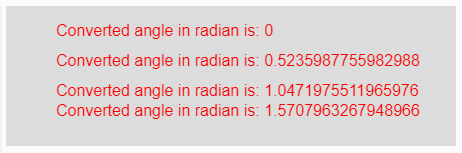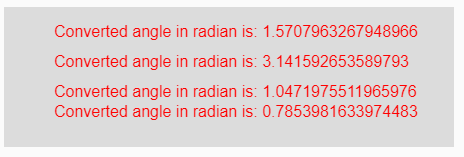# p5.js | radians() Function

• Last Updated : 22 Apr, 2019

The radians() function in p5.js is used to convert a given degree measurement value to its corresponding value in radians.

Syntax:

`radians( degrees )`

Parameters: This function accepts single parameter degrees which is to be converted into radians.

Return Value: It returns the converted radians value of angle.

Below programs illustrate the radians() function in p5.js:

Example 1: This example uses radians() function to convert a given degree to its corresponding radians.

 `function` `setup() {  ` `  `  `    ``// Creating Canvas size ` `    ``createCanvas(450, 140);  ` `}  ` ` `  `function` `draw() {  ` `      `  `    ``// Set the background color  ` `    ``background(220);  ` `    `  `    ``// Initializing some angles in degree ` `    ``let Deg1 = 0;  ` `    ``let Deg2 = 30;  ` `    ``let Deg3 = 60;  ` `    ``let Deg4 = 90;  ` `     `  `     `  `    ``// Calling to radians() function. ` `    ``let A = radians(Deg1); ` `    ``let B = radians(Deg2); ` `    ``let C = radians(Deg3); ` `    ``let D = radians(Deg4); ` `     `  `    ``// Set the size of text  ` `    ``textSize(16);  ` `      `  `    ``// Set the text color  ` `    ``fill(color(``'red'``));  ` `    `  `    ``// Getting converted angles into radians ` `    ``text(``"Converted angle in radian is: "` `+ A, 50, 30); ` `    ``text(``"Converted angle in radian is: "` `+ B, 50, 60); ` `    ``text(``"Converted angle in radian is: "` `+ C, 50, 90); ` `    ``text(``"Converted angle in radian is: "` `+ D, 50, 110); ` `}  `

Output:Example 2: This example uses radians() function to convert a given degree to its corresponding radians.

 `function` `setup() {  ` `  `  `    ``// Creating Canvas size ` `    ``createCanvas(450, 140);  ` `}  ` ` `  `function` `draw() {  ` `      `  `    ``// Set the background color  ` `    ``background(220);  ` `     `  `    ``// Calling to radians() function with different ` `    ``// degrees value as parameter ` `    ``let A = radians(90); ` `    ``let B = radians(180); ` `    ``let C = radians(60); ` `    ``let D = radians(45); ` `     `  `    ``// Set the size of text  ` `    ``textSize(16);  ` `      `  `    ``// Set the text color  ` `    ``fill(color(``'red'``));  ` `    `  `    ``// Getting converted angles in radians ` `    ``text(``"Converted angle in radian is: "` `+ A, 50, 30); ` `    ``text(``"Converted angle in radian is: "` `+ B, 50, 60); ` `    ``text(``"Converted angle in radian is: "` `+ C, 50, 90); ` `    ``text(``"Converted angle in radian is: "` `+ D, 50, 110); ` `}   `

Output: